Alert: You are not allowed to copy content or view source
Register for Maths, Science, English, GK Olympiad Exam Click here | Check Olympiad Exam Dates here | Buy Practice Papers for IMO, IOM, HEO, IOEL etc here | Login here to participate in all India free mock test on every Saturday

# Algorithms and Flowcharts in Programming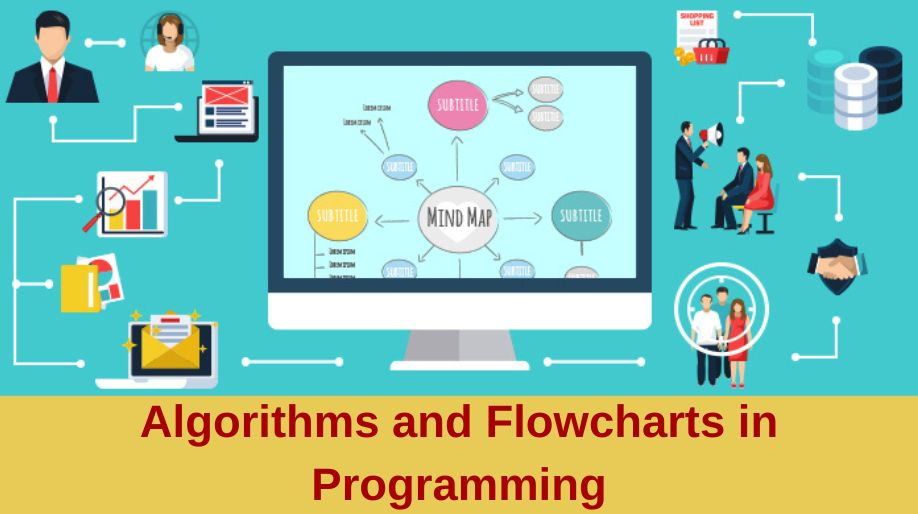Let’s learn about the algorithms and flowcharts in Programming today.

## What is a Computer Programming?

Computer programming is also known as programming. It is a process that actually leads from an original formulation of a computer problem to executable computer programs. Most important to know, a computer program is usually written by a computer programmer in a programming language itself. It is important because it defines the relationship, grammar and semantics which ultimately helps the programmer to communicate effectively with the machines that they program.

## A typical programming task can be divided into two phases:

1. Problem-solving phase
• Produce an ordered sequence of steps that describe the solution of the problem
• This sequence of steps is called an algorithm
1. Implementation phase
• Implement the program in some programming language

Algorithms and flowcharts are two different tools used for creating new programs. So, knowing both of them well in advance is a great idea to start programming easily.

## Algorithm

An algorithm is a well-defined procedure that allows a computer to solve a problem. In computer science, an algorithm is a step-by-step procedure for calculations. Algorithms are used for calculation, data processing, and automated reasoning. Basically, it can be presented by natural languages, pseudo code and flowcharts, etc.

Characteristics of a Good Algorithm

1. Precision: The steps are precisely defined in an algorithm to give accurate results.
1. Uniqueness: Results of each step are unique and defined. And, the result depends on the input and the result of the previous step.
1. Finiteness: The algorithm ultimately stops itself when a finite number of instructions are executed.
1. Easy modifications: In algorithm, you can easily make modifications that make the process easier.
1. Easy and simple to understand: The steps are easy and understandable to the non-programmers too.

## Solved Examples

Example 1: Print 1 to 10:

Algorithm:

Step 1: Initialize X as 0,

Step 2: Increment X by 1,

Step 3: Print X,

Step 4: If X is less than 10 then you need to repeat the step 2.

Example 2: Convert Temperature from Fahrenheit () to Celsius ()

Algorithm:

Step 1: Read temperature in Fahrenheit,

Step 2: Calculate temperature with formula C=5/9* (F-32),

Step 3: Print C,

## Flowchart

A schematic representation of a sequence of operations, as in a manufacturing process or computer program is known as a flowchart or we can say flowchart is a graphical representation of the sequence of operations in an information system or program.

Basic Characteristics of Flowchart

• It shows the logic behind the algorithm.
• It literally emphasizes individual steps and their interconnections.
• It clearly illustrates the flow of programming techniques, they are a valuable tool in the education of programmers
• control flow from one action to the next action.

Types of Flowchart

There are four general types of a flowchart

1. Document flowcharts, which shows controls over a document flow through a defined system.
1. Data flowcharts, which shows controls over a data flow in a system.
1. System flowcharts, which shows controls at a physical or resource level.
1. Program flowchart, which shows the controls in a program within a system.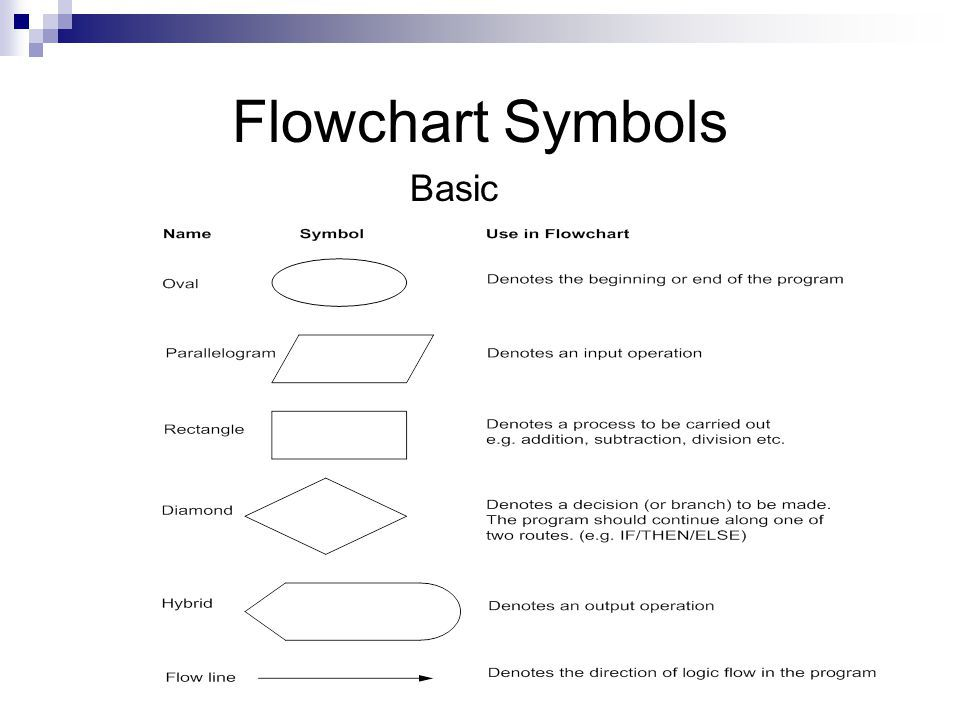## Pseudocode in Programming

• The pseudocode in programming is generally a detailed yet readable description of what a computer program or algorithm must do.
• The main important thing about pseudocode is that it is not in the programming language, rather it is in formally style natural language.
• Thus, enabling easy work. Sometimes, pseudocode is used as an important and detailed step in the process of developing a program.

Both algorithm and pseudocode are two different things. But, you can base your pseudocode on an algorithm that is the thing,  They are used to plan out programs.

## Solved Examples

Example 1: Draw a flowchart to convert the length in feet to centinmeter.

Flowchart:

Here, Pseudocode is used.

1. Input the length in feet (Lft)
2. Calculate the length in cm (Lcm) by multiplying LFT with 30
3. Print length in cm (LCM)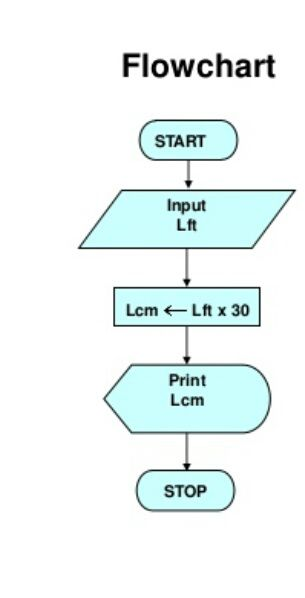Example 2: Draw a flowchart that will read the two sides of a rectangle and calculate its area.

Flowchart:

Here Pseudocode is used.

1. Input the width (W) and length (L) of a rectangle.
2. Calculate the area (A) by multiplying L with W.
3. Print A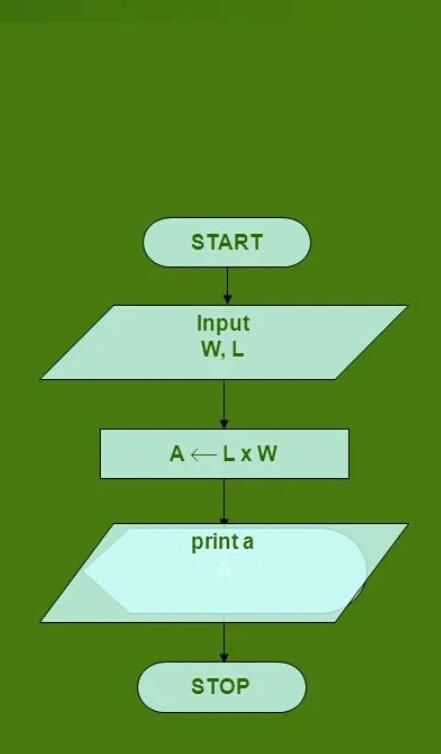## Recap

• The basic knowledge about computer programming and basic two tools used in it; algorithm and flowchart.
• An algorithm is a well-defined procedure that allows a computer to solve a problem well explained with the help of examples.
• Characteristics of a good algorithm.
• A schematic representation of a sequence of operations, as in a manufacturing process or computer program is known as a flowchart, The basic characteristics and its types.
• Basic Flowchart symbols and their use.
• The pseudocode in programming is generally a detailed yet readable description of what a computer program or algorithm must do. Different from an algorithm.
• Flowchart well explained through examples having pseudocode.

## Quiz for Algorithms and Flowcharts in Programming

 Q.1 Which symbol is used to indicate the flow of logic by connecting symbols. a) Flow Line b) Terminal c) Input/Output d) Processing
 Q.2 Which of the following is used for arithmetic operations and data manipulations? a) Flow Line b) Processing c) Decision d) On-Page
 Q.3 Which symbol is used to indicate stop/start of the program? a) Terminal b) Processing c) Decision d) Input/Output
 Q.4 How many types of flowcharts symbols are there? a) 10 b) 9 c) 7 d) 6
 Q.5 int x=10; int y=20, what type of examples are these? a) Relational b) Binary c) Logical d) Assignment
 Q.6 What is the output of the following statement int x=10; x=x+2; a) x'='8 b) x'='10 c) x'='12 d) x'='2
 Q.7 Which of the following is a flow line symbol? a)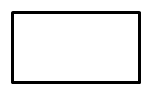a b)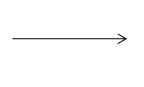b c)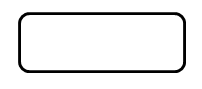c d)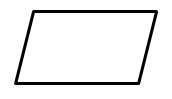d
 Q.8 Which of the following is a Terminal symbol? a)a b)b c)c d)d
 Q.9 Which of the following is a Manual Input symbol? a)a b)b c)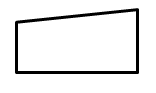c d)d
 Q.10 The output of the above flowchart is: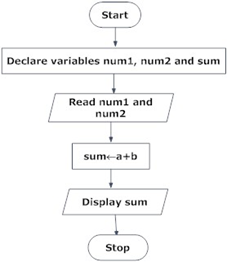a) Subtraction of Two Numbers b) Addition of Two Numbers c) Fibonacci d) Factorial